# Puzzle 29 | (Car Wheel Puzzle)

• Difficulty Level : Easy
• Last Updated : 13 Jan, 2022

Puzzle: A car has 4 tyres and 1 spare tyre. Each tyre can travel a maximum distance of 20000 miles before wearing off. What is the maximum distance the car can travel before you are forced to buy a new tyre? You are allowed to change tyres (using the spare tyre) an unlimited number of times.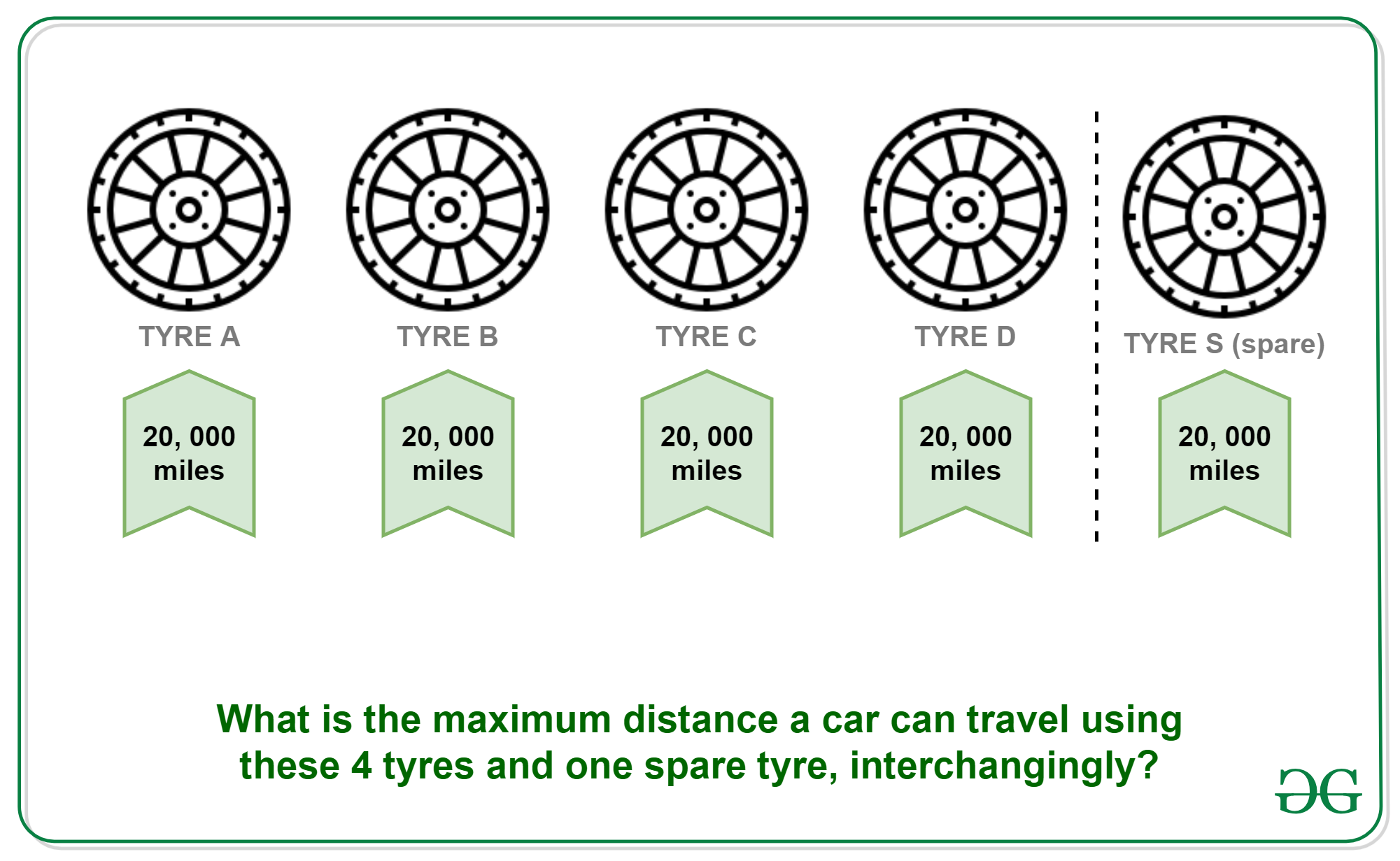Solution: Divide the lifetime of the spare tire into 4 equal part i.e., 5000 and swap it at each completion of 5000 miles distance.
Let four tyres be named as A, B, C and D and spare tyre be S.

1. 5000 KMs: Replace A with S. Remaining distances (A, B, C, D, S) : 15000, 15000, 15000, 15000, 20000.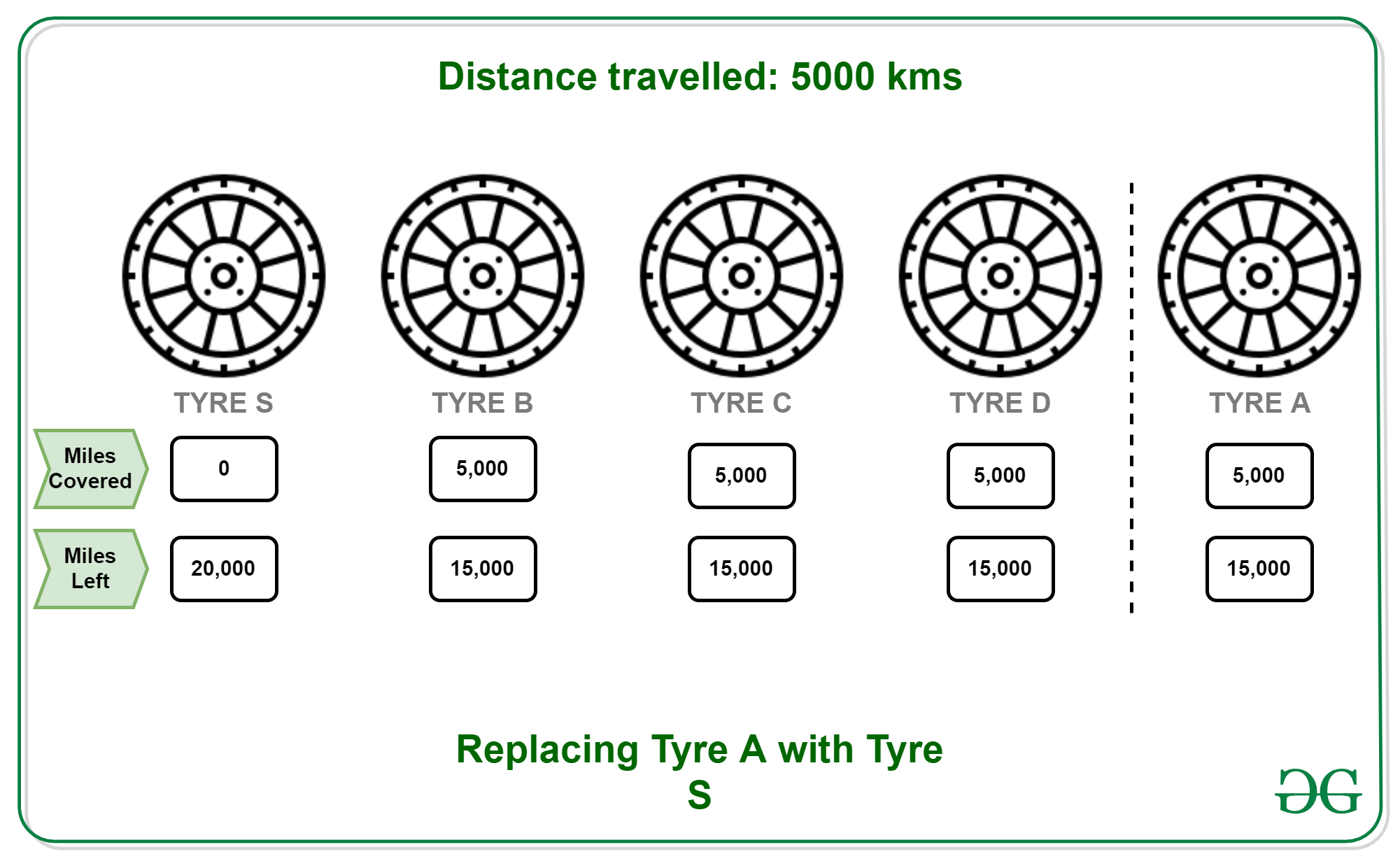1. 10000 KMs: Put A back to its original position and replace B with S. Remaining distances (A, B, C, D, S) : 15000, 10000, 10000, 10000, 15000.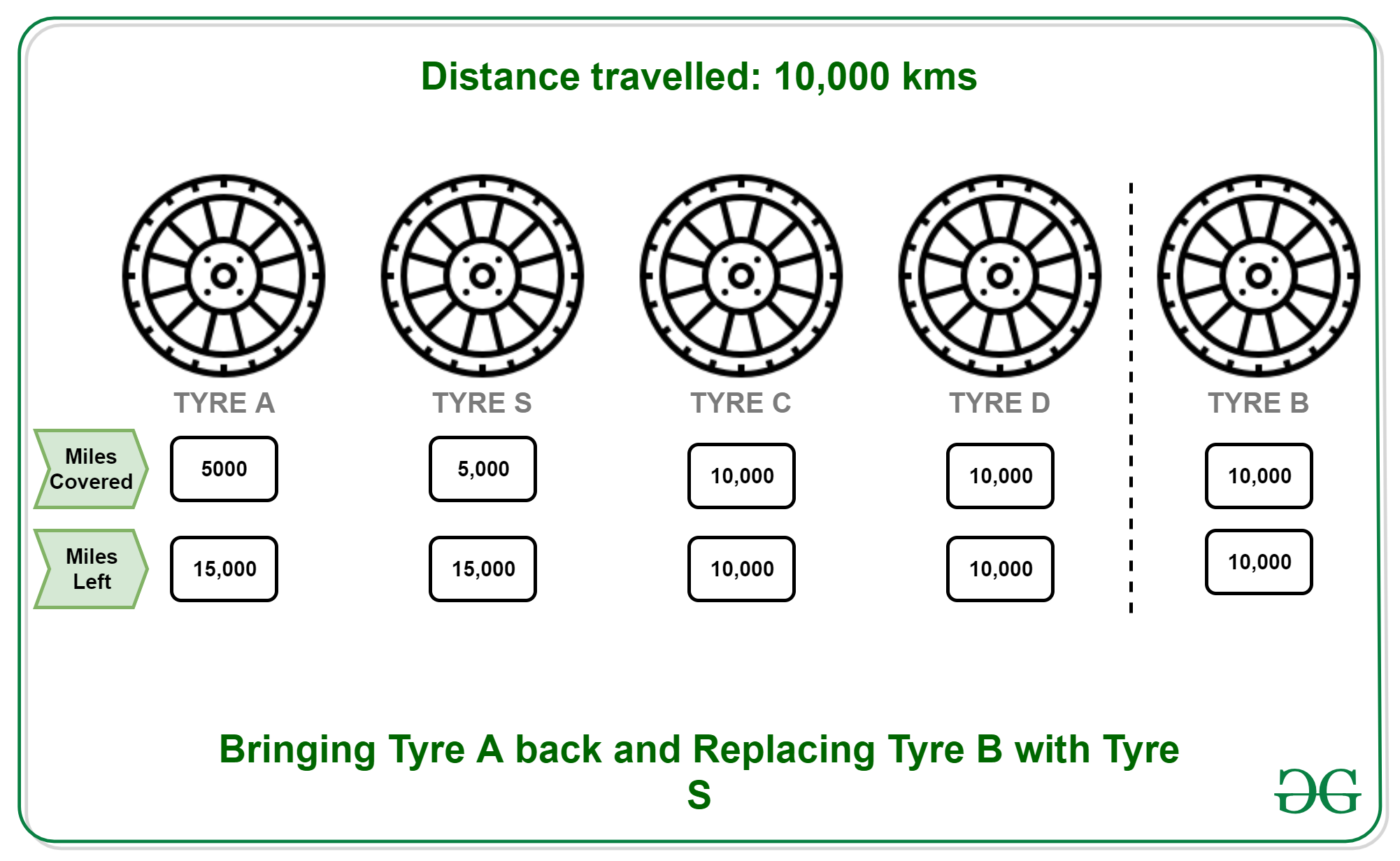1. 15000 KMs: Put B back to its original position and replace C with S. Remaining distances (A, B, C, D, S) : 10000, 10000, 5000, 5000, 10000.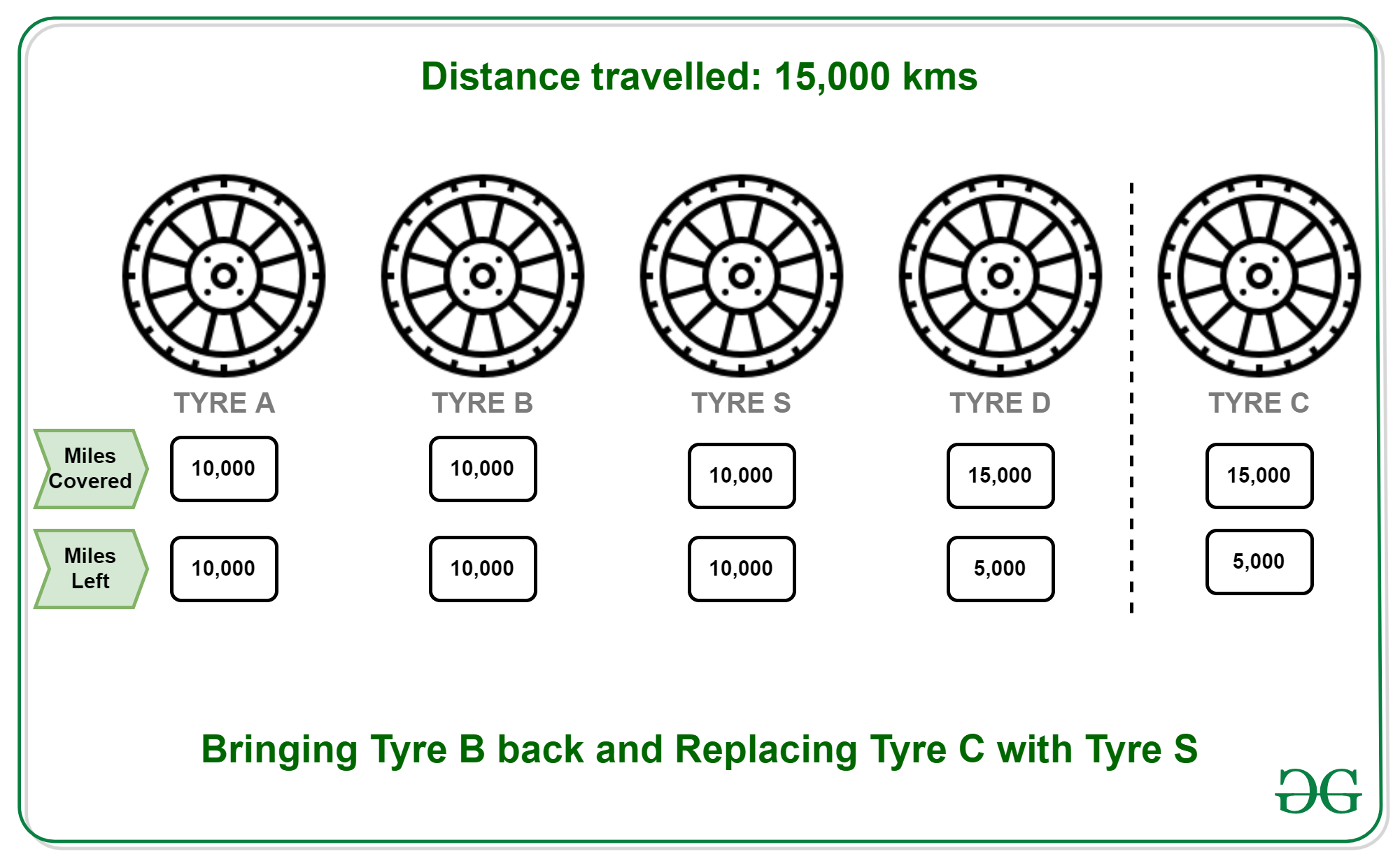1. 20000 KMs: Put C back to its original position and replace D with S. Remaining distances (A, B, C, D, S) : 5000, 5000, 5000, 0, 5000.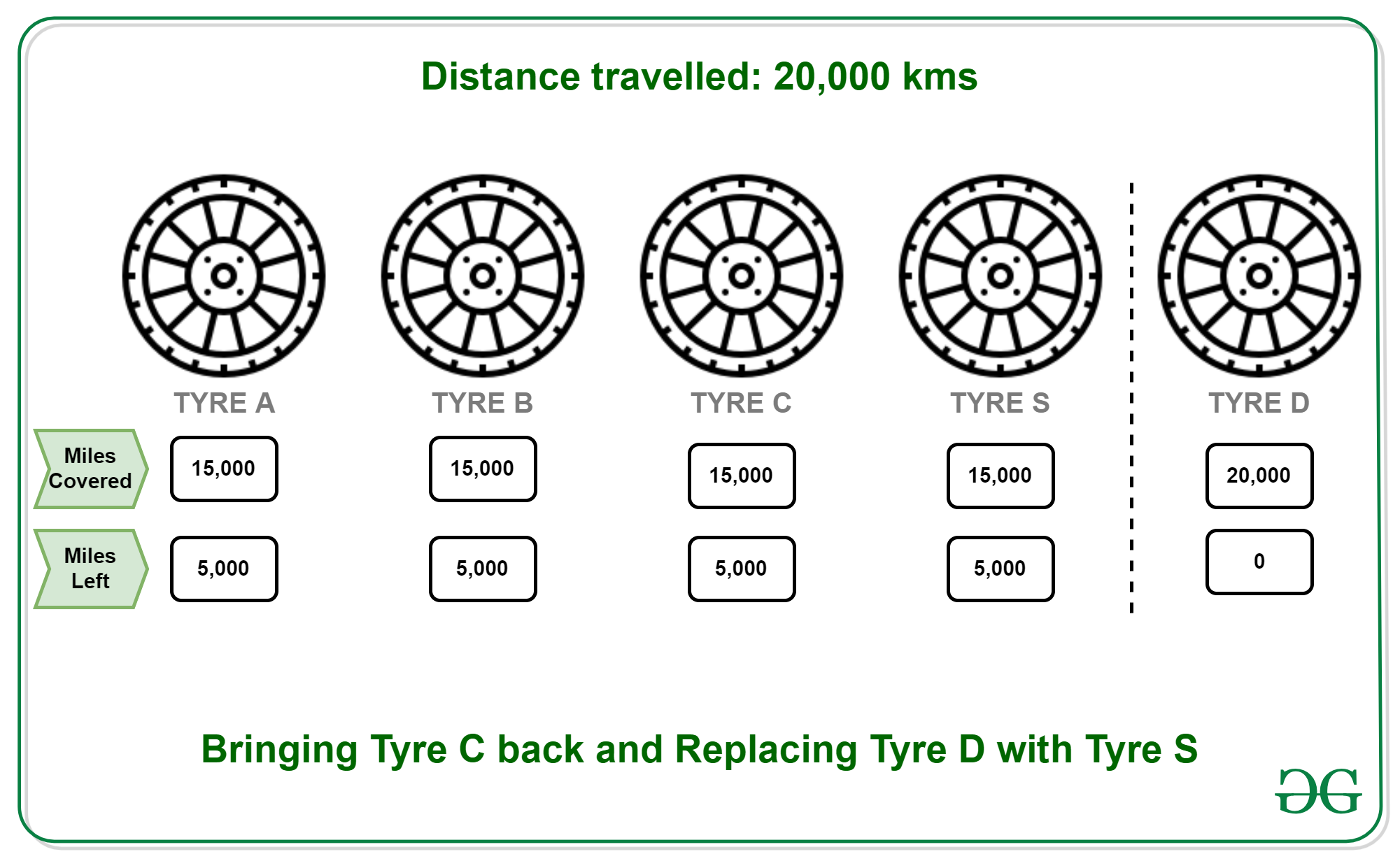1. 25000 KMs: Every tyre is now worn out completely.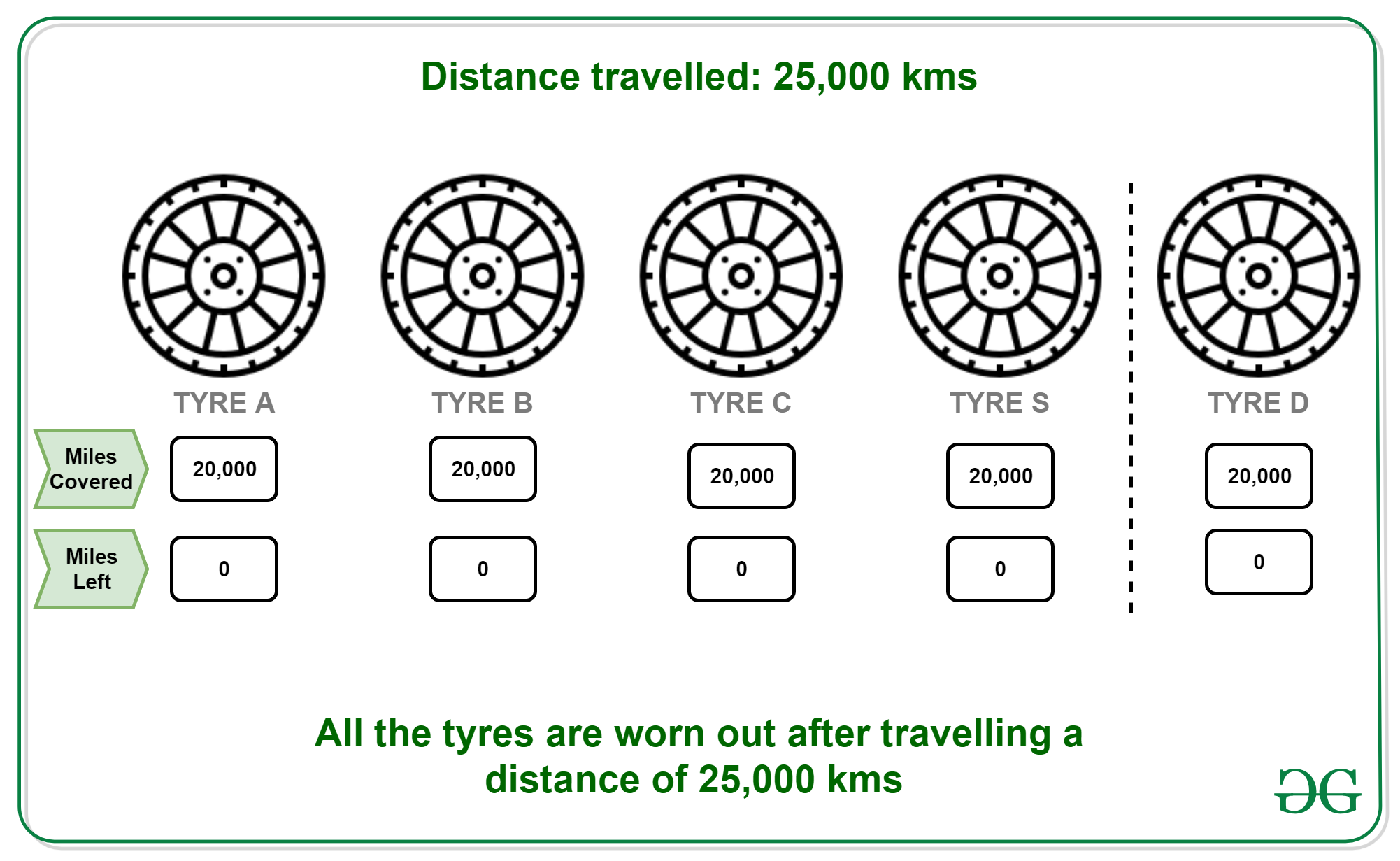All tyres are used to their full strength.

### Related Amazon interview question

There are n pencils, each having l length. Each can write 4 kilometres. After writing 4 kilometres it has l/4 length. One can join 4 pencils which are having l/4 length and can make 1 pencil. One can’t make pencil of pieces if remaining pieces are 3 or 2 or 1 in number but one can include these remaining pieces whenever needed.
Write a relation independent of l, length of the given pencil, for how much one can write from n pencils.
Examples:

```Input: 4
Output: 20```

Recursive Approach:
Suppose we use 3 pencils that will 12 and generate 3 used pencils, now if the remaining pencils are greater than zero at least 1 unused pencil can be used with those 3 unused to write 4 and that will generate 1 more unused pencil. This will keep repeating.

```if(n-3 >= 1){
f(n) = f(n-3) + 12 + 4
}
else{
// Used pencil that cannot be used
f(n) = 4*n
}```

Below is the implementation of the above approach:

## C

 `int` `count(``int` `n)``{``    ``if` `(n < 4) {``        ``return` `(n * 4);``    ``}``    ``else` `{``        ``return` `(16 + count(n - 3));``    ``}``}`

Mathematical Approach O(1):
Above relation can be optimized in O(1)

```// x is max no of time we can
//subtract 3 without n-3 <= 3
n - 3*x <= 3
x  >  (n-3)/3
i.e. n/3 - 1 if it divides exactly
else n/3```

Below is the implementation of the above approach:

## CPP

 `// C++ program to find relation independent of l``// length of the given pencil, for how much one``// can write from n pencils.` `#include ``using` `namespace` `std;` `// Function to find no of pencils``int` `count(``int` `n)``{``    ``int` `x = (n / 3) - 1;``    ``if` `(n % 3) {``        ``x++;``    ``}``    ``return` `(4 * x + 4 * n);``}` `// Driver function``int` `main()``{``    ``int` `n = 5;``    ``cout << count(n) << endl;``}`

## Java

 `// Java program to find relation independent of l``// length of the given pencil, for how much one``// can write from n pencils.``class` `GFG``{` `// Function to find no of pencils``static` `int` `count(``int` `n)``{``    ``int` `x = (n / ``3``) - ``1``;``    ``if` `(n % ``3` `> ``0``)``    ``{``        ``x++;``    ``}``    ``return` `(``4` `* x + ``4` `* n);``}` `// Driver code``public` `static` `void` `main(String[] args)``{``    ``int` `n = ``5``;``    ``System.out.print(count(n) +``"\n"``);``}``}` `// This code is contributed by PrinciRaj1992`

## Python3

 `# Python3 program to find relation independent of l``# length of the given pencil, for how much one``# can write from n pencils.` `# Function to find no of pencils``def` `count(n):``    ``x ``=` `(n ``/``/` `3``) ``-` `1``;``    ``if` `(n ``%` `3` `> ``0``):``        ``x``+``=``1``;``    ` `    ``return` `(``4` `*` `x ``+` `4` `*` `n);` `# Driver code``if` `__name__ ``=``=` `'__main__'``:``    ``n ``=` `5``;``    ``print``(count(n));``    ` `# This code is contributed by PrinciRaj1992`

## C#

 `// C# program to find relation independent of l``// length of the given pencil, for how much one``// can write from n pencils.``using` `System;` `class` `GFG``{` `// Function to find no of pencils``static` `int` `count(``int` `n)``{``    ``int` `x = (n / 3) - 1;``    ``if` `(n % 3 > 0)``    ``{``        ``x++;``    ``}``    ``return` `(4 * x + 4 * n);``}` `// Driver code``public` `static` `void` `Main(String[] args)``{``    ``int` `n = 5;``    ``Console.Write(count(n) +``"\n"``);``}``}` `// This code is contributed by 29AjayKumar`

## Javascript

 ``
Output:
`24`

My Personal Notes arrow_drop_up